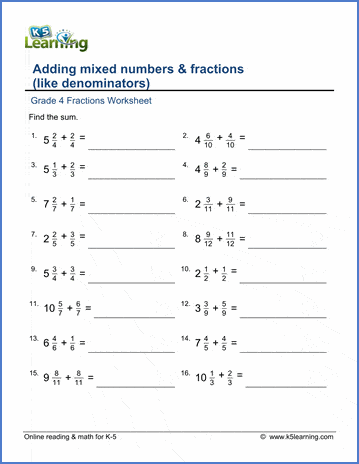# Adding mixed numbers & fractions

## Worksheets: Adding mixed numbers & fractions (like denominators)

Below are six versions of our grade 4 fractions worksheet on adding mixed numbers to fractions with like denominators.  Answers are given as mixed numbers with no improper fractions. These worksheets are pdf files.## More fractions worksheets

Explore all of our fractions worksheets, from dividing shapes into "equal parts" to multiplying and dividing improper fractions and mixed numbers.

## What is K5?

K5 Learning offers reading and math worksheets, workbooks and an online reading and math program for kids in kindergarten to grade 5.  We help your children build good study habits and excel in school.